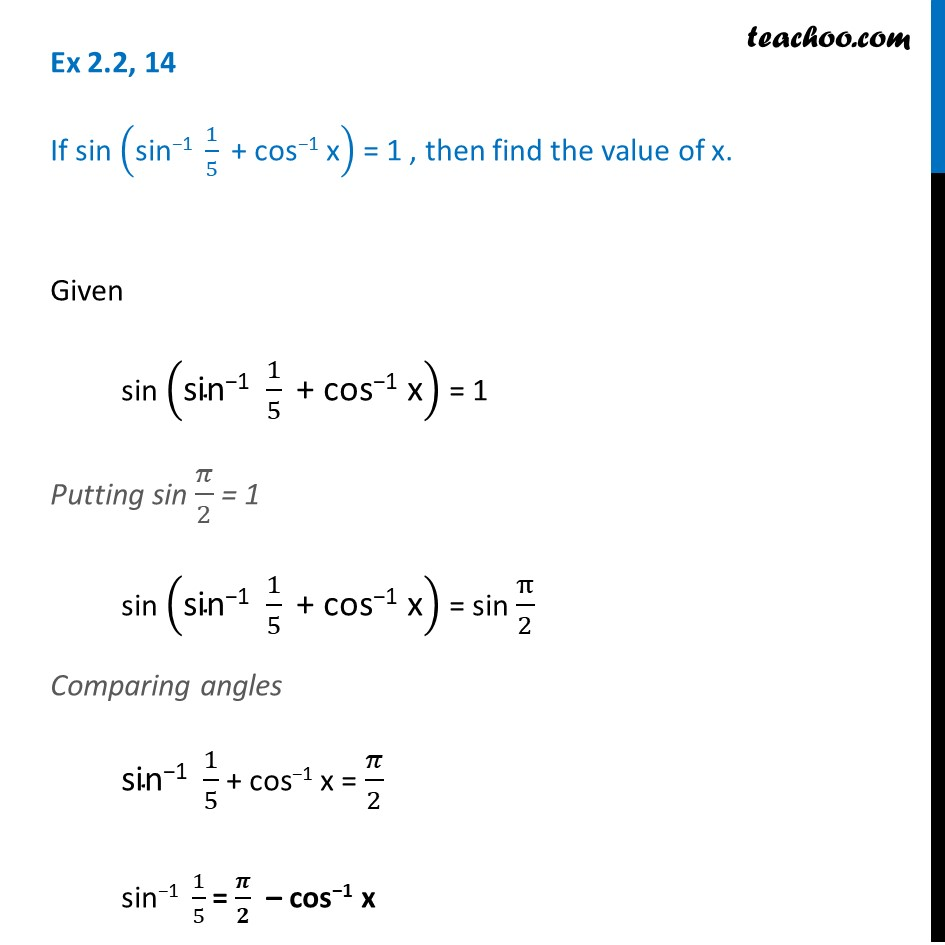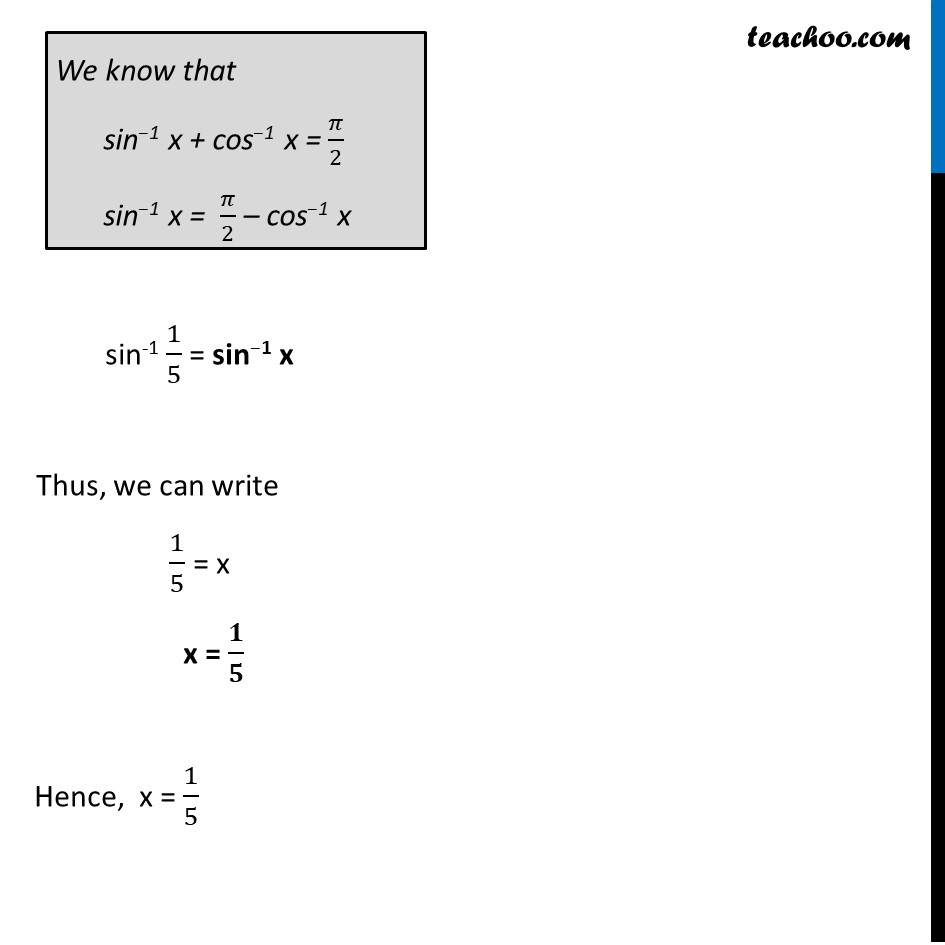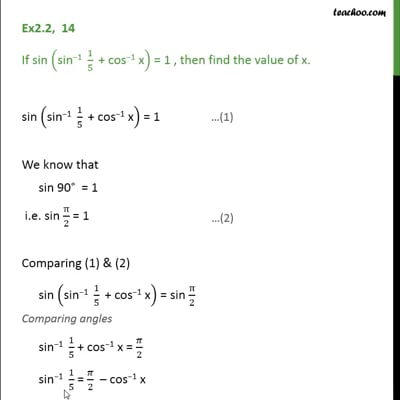Ex 2.2

Chapter 2 Class 12 Inverse Trigonometric Functions
Serial order wiseThis video is only available for Teachoo black users

Learn in your speed, with individual attention - Teachoo Maths 1-on-1 Class

### Transcript

Question 5 If sin ("sin−1 " 1/5 " + cos−1 x" ) = 1 , then find the value of x. Given sin ("sin−1 " 1/5 " + cos−1 x" ) = 1 Putting sin 𝜋/2 = 1 sin ("sin−1 " 1/5 " + cos−1 x" ) = sin π/2 Comparing angles "sin−1 " 1/5 + "cos−1 x" = 𝜋/2 "sin−1 " 1/5 = 𝝅/𝟐 – "cos−1 x" We know that sin"−"1 x + cos"−"1 x = 𝜋/2 sin"−"1 x = 𝜋/2 – cos"−"1 x sin-1 1/5 = sin"−"1 x Thus, we can write 1/5 = x x = 𝟏/𝟓 Hence, x = 1/5